top of page

# Light – Reflection and Refraction

## What is Light?

We are able to see the objects when light is reflected back from them. Therefore, light is a form of energy that allows us to see. Light is a form of electromagnetic radiation and thus does not require any medium to travel.

We are able to see through a transparent medium when light passes through it.

When light is absorbed completely by an object we are unable to see it as no light bounces off it.

### Nature and Properties of Light

1. Light has dual nature: Light particles can behave as both particles as well as waves.

• Particle Nature of Light: For example when light falls on a photoelectric medium, it ejects some electrons. This is called as photoelectric effect. This could be explained only by particle nature of light.

• Wave Nature of Light: When light passes through a medium and it encounters very small particles, it tends to bend around them. This phenomenon is called as dispersion of light and could be only explained if light were to behave like a wave.

One famous experiment that proves the duality of lights is the Young’s Double Slit Experiment.

2. Light Travels in a Straight Line: The phenomenon of reflection and refraction of light can be explained by the property of the light that it travels in a straight line.

3. The maximum speed of light is 3 x 108 m/s.

4. When light falls on an object it may be reflected, refracted or absorbed.

## Reflection of Light

When light falls on a polished surface it bounces back from it and this phenomenon is called as reflection of light.

### Laws of Reflection

1. The angle of incidence is equal to the angle of reflection

2. The incident ray, the normal to the mirror at the point of incidence and the reflected ray, all lie in the same plane.

These laws of reflection are valid for all kinds of reflecting surfaces including spherical surfaces.

### Laws of Reflection of Light - Experiment

#### What is Fermat’s Theorem?

1. The principle of least time: Light always takes the quickest path between any two points (which may not be the shortest path).

2. Rectilinear propagation of light and the law of reflection [∠i=∠r] can be validated by Fermat’s principle of least time.

#### Reflection of Light on a Plane Mirror### What are the characteristics of Image formed by a plane mirror?

• It is always virtual and erect.

• The size of the image is equal to that of the object.

• The image formed is as far behind the mirror as the object is in front of it.

• It is laterally inverted. i.e. the right side of the image appears to be left and vice versa.

### Difference between Real and Virtual Image

 REAL IMAGE VIRTUAL IMAGE It is formed when the ray of light actuallymeet It is formed when rays of light appear tomeet It can be obtained on a screen ​It cannot be obtained on a screen It is always inverted It is always erect Example : Image formed on a cinemascreen Example : Image formed in a planemirror

## Spherical MirrorsPrincipal axis: The line joining the pole and center of curvature.

Pole (P): The centre of the spherical mirror.

Aperture (MN): It is the effective diameter of the spherical mirror.

Center of Curvature (C): The centre of the hollow glass sphere of which the mirror was a part.

Radius of Curvature (R): The distance between the pole and the centre of curvature.

Focus (F): The point on principal axis where all the parallel light rays actually meet or appear to meet after reflection.

Focal length (f): The distance between the pole and the focus.

## Convex Mirrorsi) Reflecting Surface is Curved Outwards

ii)It is also called as diverging mirror

iii)It has positive focal length as focus lies on the positive side of the Pole of the Mirror

iv) It always forms a virtual image

### Concave Mirrorsi) Reflecting Surface is Curved Inwards

ii) It is also called as converging mirror

iii) It has negative focal length as focus

lies on

the left side of the Pole of the Mirror

iv) It can form both real and inverted

images.Rules for making ray diagrams for spherical mirrors

(i) A ray parallel to the principal axis will pass through the principal focus, after reflection.(ii) A ray passing through the principal focus of concave mirror will emerge parallel to principal axis after reflection.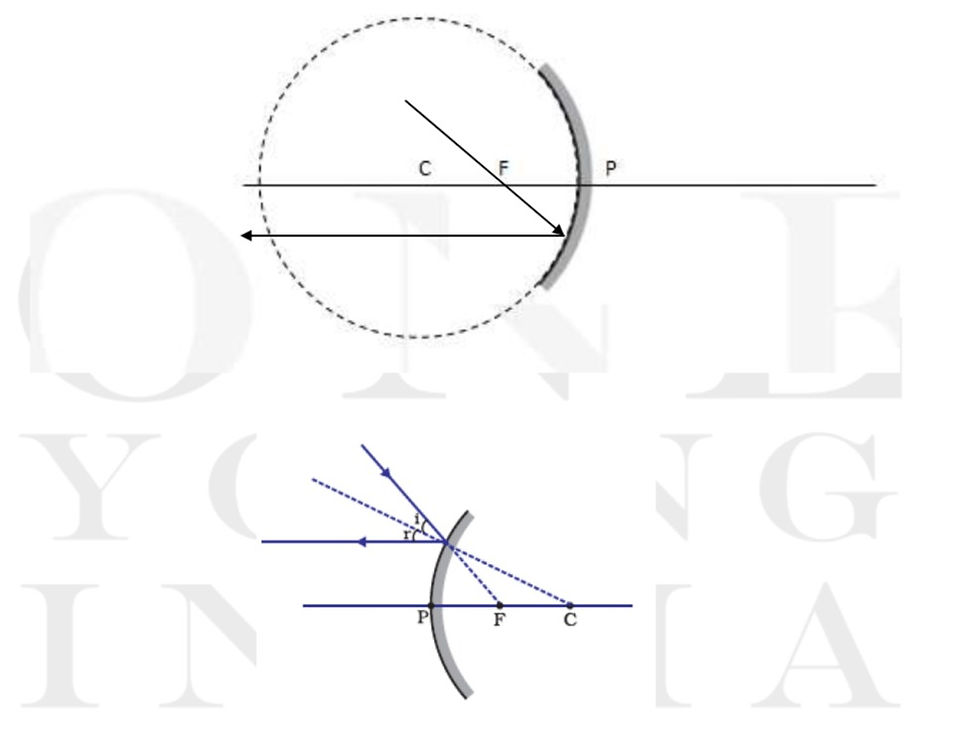(iii) A ray of light passing through the centre of curvature of a concave mirror is reflected back along the same path as it is a normally incident ray.(iv) A ray incident obliquely to the principal axis of a concave mirror is reflected obliquely making equal angle.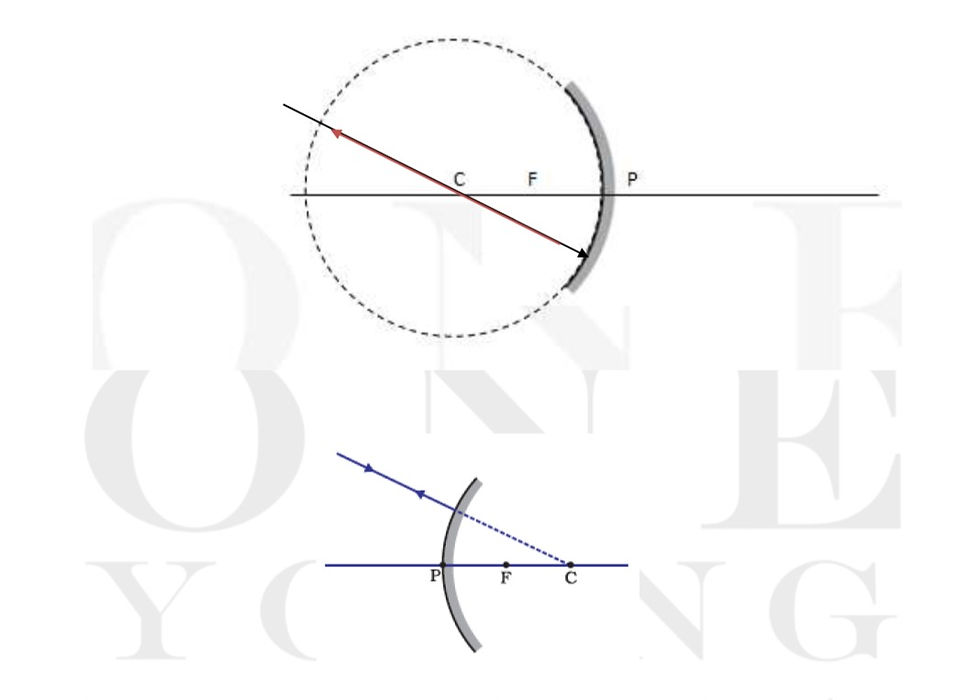#### Image Formation by Concave Mirrors

 Position of Object Position of Image Size of Image Nature of Image At Infinity At Focus Point Sized or Highly Diminished Real and Inverted Beyond C Between F and C Highly Diminished Real and Inverted At C At C Same Size Real and Inverted Between C and F Beyond C Enlarged Real and Inverted At F At Infinity Highly Enlarged Real and Inverted Between F and P Behind the mirror Enlarged Virtual and Erect

Case I - Object At InfinityCase II - Object Beyond CCase III – Object at CCase IV - Object Between C and F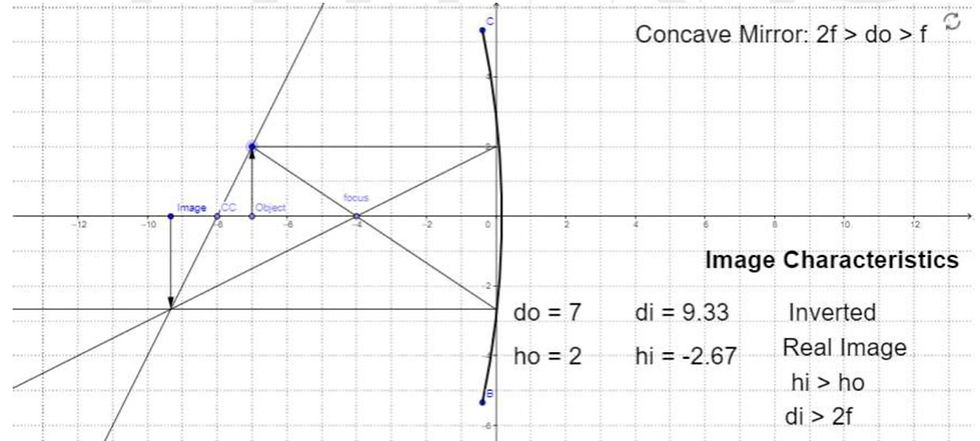Case V - Object At FTheoretically, Image will be formed at Infinity, but since both the rays are parallel they will

not interest at any visible point.

Case VI - Object Between F and PUses of concave mirrors

-Used in torches, search-lights and vehicles headlights to get powerful parallel beams of light.

-Used as shaving mirrors to see a larger image of the face.

-The dentists use concave mirrors to see large images of the teeth of patients.

-Large concave mirrors are used to concentrate sunlight to produce heat in solar furnace

#### Images Formation by Convex Mirrors

 Position of Object Position of Image Size of Image Nature of Image At Infinity At Focus, behind the mirror Point Sized or Highly Diminished Virtual and Erect Between Infinity and Pole of the mirror Between P and F, behind the mirror Diminished Virtual and Erect

Case I - Object at InfinityCase II - Object Between Infinity and PoleUses of convex mirrors

- Used as rearview (wing) mirrors in vehicles enabling the driver to have a wider view of traffic behind him/her to facilitate safe driving.

- They are used around corners to provide a wide view.

New Cartesian Sign Convention for Spherical Mirrors

1. The principal axis is taken as the x-axis and the pole of the mirror is taken as the origin.

2. The object is always placed on the left hand side of the mirror. (Object is on the negative X axis)

3. The pole of the mirror is taken as the origin and all distances are measured from the Pole.

4. Distances to the right are taken as positive and distances to the left are taken as negative

5. Distances measured perpendicular to and above the principal axis are taken as positive

(along the + y axis)

6. Distances measured perpendicular to and below the principal axis are taken as positive

(along the - y axis)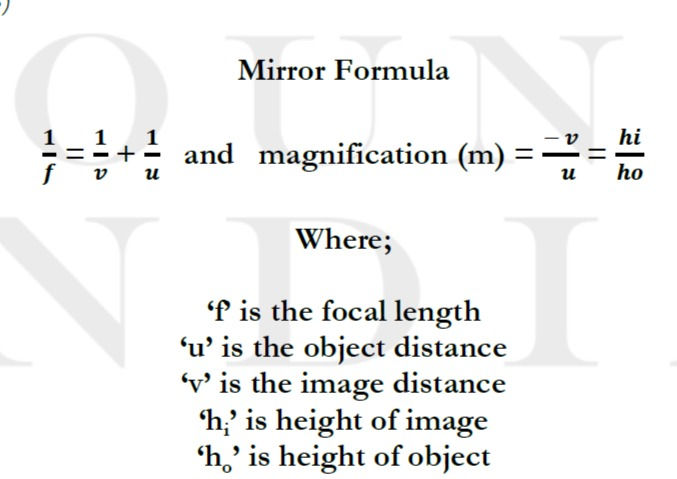Note:

Focal Length of a Concave mirror is Negative

Focal Length of a Convex mirror is Positive

Object distance is always taken as Negative

If ‘m’ is negative , image is real and inverted

If ‘m’ is positive, image is virtual and erect

### Refraction of Light

When lights travels obliquely from one medium to another, the direction of propagation of light in the second medium changes. This phenomenon is known as refraction of light.

What causes refraction?

The change in speed of light as it travels from one medium to another and the property of light that principle that light always takes the quickest path leads to change in the direction of light..

Some examples of refraction:(i) A filled swimming pool appear shallower

(ii) A pencil partially immersed in water appears to be bent at the interface of water and air.

(iii) Letters of a book appear to be raised when seen through a glass slab.

Refraction through glass slab:

Light Travels Obliquely = DeviationLight Travels Straight = No Deviation1. The extent of bending of ray of light at the opposite parallel faces of rectangular glass slab is equal and opposite, so the ray emerges parallel to incident ray.

2 Lateral displacement depends on :

(a) Refractive index of glass slab

(b) Thickness of the glass slab

#### Laws of refraction of light.

(i) The incident ray, the refracted ray and the normal to the interface of two transparent media at the point of incidence, all lie in the same plane.

### Snell’s law of refraction

(ii) The ratio of sine of angle of incidence to the sine of angle of refraction is a constant, for the light of a given colour and for the given pair of media.

(This is true for angle 0 < i < 90o)

If i is the angle of incidence and r is the angle of refraction, then:Absolute Refractive Index: Refractive index of a medium with respect to vacuum or air.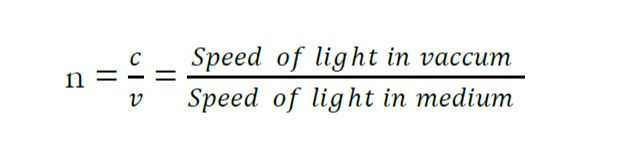A medium with higher absolute refractive index is optically denser while one with lower absolute refractive index is optically rarer.

Refractive index of a medium does not depend on physical density.

When an object travels from a rarer from a denser medium, it bends towards the normal:When an object travels from a rarer from a denser medium, it bends towards the normal:### Spherical lens:

A transparent medium bound by two surfaces, of which one or both surfaces are curved.Focal length of convex lens = Positive

Focal length of concave lens = Negative

#### Rules for image formation by spherical lenses

(i) A ray of light parallel to principal axis of a convex lens always pass through the focus on the other side of the lens.

A ray of light parallel to the principal axis appear to diverge from the principal focus located on the same side of the lens.ii) A ray of light passing through the principal focus of a convex lens will emerge parallel to

principal axis after refraction.

A ray of light appearing to meet at the principal focus of a concave lens will emerge parallel to principal axis.iii) A ray of light passing through the optical center will emerge without any deviation.

A ray of light passing through the optical centre of a lens will emerge without any deviation.##### Image Formation by Convex Lens
 Position of Object Position of Image Size of Image Nature of Image At Infinity At F2 Point Sized or Highly Diminished Real and Inverted Beyond 2F1 Between F2 and 2F2 Highly Diminished Real and Inverted At 2F1 At 2F2 Same Size Real and Inverted Between 2F1 and F1 Beyond 2F2 Enlarged Real and Inverted At F1 At Infinity Highly Enlarged Real and Inverted Between F1 and O On the same side of the lease as the object Enlarged Virtual and Erect

Case I - Object at InfinityCase II - Object Beyond 2F1Case III - Object at 2F1Case IV - Object between 2F1 and F1Case V - Object at F1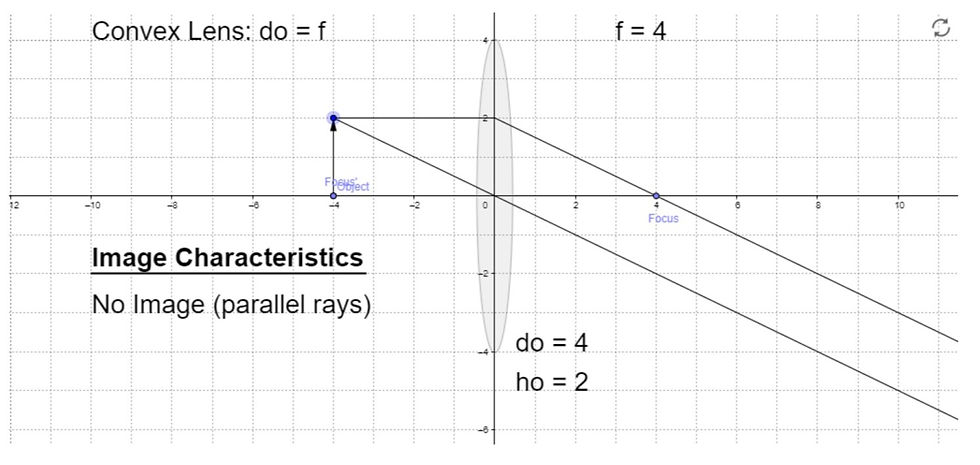Theoretically, image will be formed at infinity, but since both the rays are parallel they will not intersect at any visible point.

Case VI - Object between F1 and O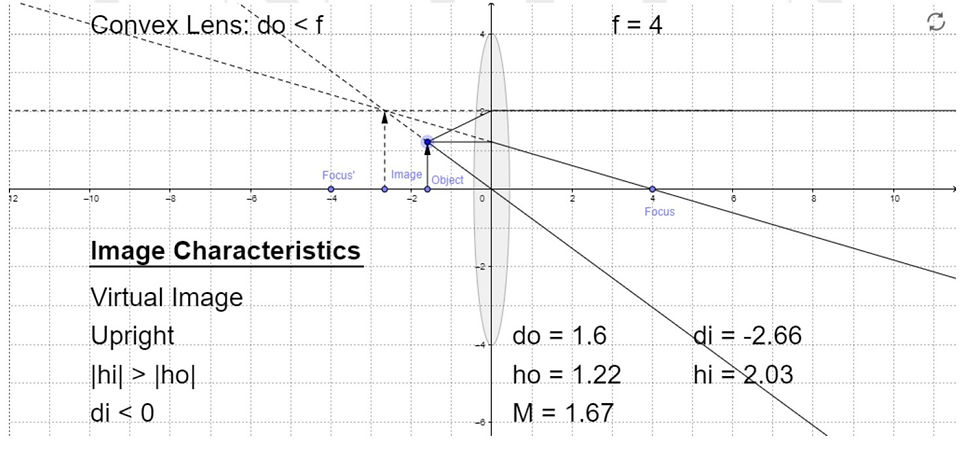##### Image Formation by Concave Lens
 Position of Object Position of Image Size of Image Nature of Image At Infinity At F1 Point Sized or Highly Diminished Virtual and Erect Between Infinity and Optical Centre of the Lens Between F1 and O Diminished Virtual and Erect

Case I - Object at InfinityCase II - Object between Infinity and Optical center of the Lens#### Power of a Lens

It is defined as the reciprocal of focal length in meter. the degree of convergence or divergence of light rays is expressed in terms of power.

SI unit of Power = dioptre (D)1 dioptre is the power of lens whose focal length is one meter.See All